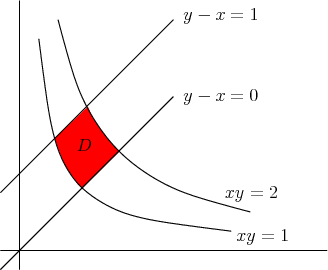# Math Insight

### Image: Example region for changing variables in a double integralTo calculate the double integral over the pictured region $\dlr$, one could use the change of variables $\cvarfv = y-x$ and $\cvarsv=xy$.

Image file: double_integral_change_variable_example.png